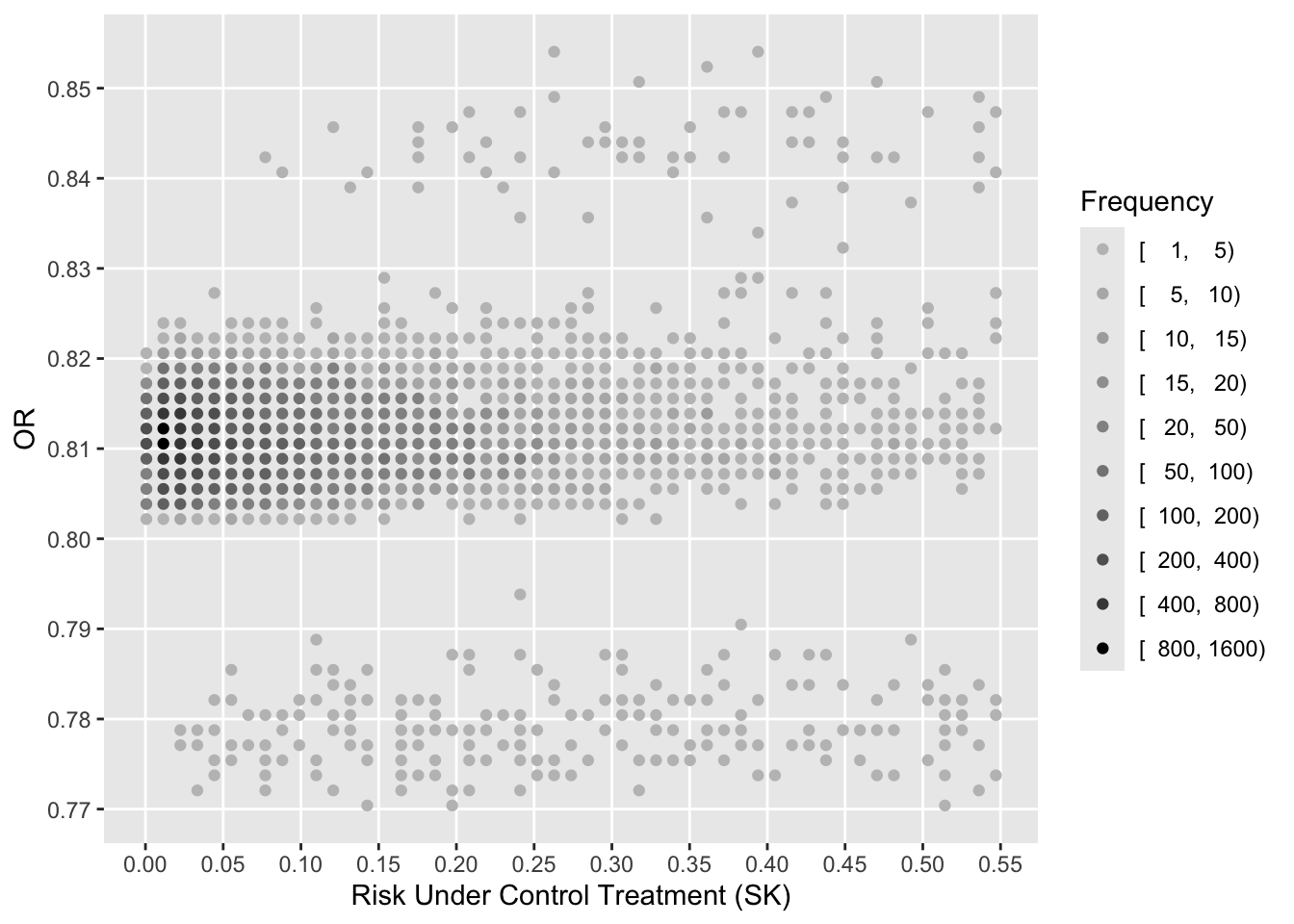# Assessing Heterogeneity of Treatment Effect, Estimating Patient-Specific Efficacy, and Studying Variation in Odds ratios, Risk Ratios, and Risk Differences

RCT
generalizability
medicine
metrics
personalized-medicine
prediction
subgroup
accuracy-score
2019
This article shows an example formally testing for heterogeneity of treatment effect in the GUSTO-I trial, shows how to use penalized estimation to obtain patient-specific efficacy, and studies variation across patients in three measures of treatment effect.
Author
Affiliation

Vanderbilt University
School of Medicine
Department of Biostatistics

Published

March 25, 2019

## Background

Heterogeneity of treatment effect (HTE), better called differential treatment effect, is variation in a measure of treatment effect on a scale for which it is mathematically possible that such variation be absent even if the treatment has a nonzero effect. The most commonly used scales for measuring HTE are

• the original scale for continuous response variables that are properly transformed, in which case the treatment effect is the (adjusted) difference in means
• odds ratios, for binary or ordinal outcomes
• hazard ratios, for time-to-event outcomes

Even though the more complex hazard ratio seems to be well accepted as a summary measure of treatment effect in a time-to-event randomized clinical trial (RCT), there is still a good deal of resistence to odds ratios (OR) from some clinical researchers. This resistence is difficult to understand, although it is clear that ORs are more difficult to understand than risk ratios (RR) or absolute risk reduction (ARR; risk difference). The reasons for choosing ORs are:

• ORs come directly from logistic models, and logistic models are as likely as any model to fit patterns leading to binary responses. This is primarily because the logistic model places no restrictions on the regression coefficients.
• ORs are capable of being constant over a range of baseline risk all the way from 0 to 1.0.
• In the multitude of forest plots present in journal articles depicting RCT results, the constancy of ORs over patient types is impressive.
• Unlike RRs, ORs are invariant to the choice of the “event” vs. the “no event”. If you interchange event and non-event you would get the reciprocal of the original OR, but the RR would change arbitrarily.

RR and ARR are not capable of being constant. For example, a risk factor that doubles the risk of an outcome can only apply to a patient having a baseline risk of 0.5 or less, otherwise the risk with the risk factor would exceed 1.0. A treatment that lowers the risk of an outcome of 0.04 cannot apply to a patient with a baseline risk of 0.02.

The purposes of this article are to show how

• a rigorous test for HTE in the frequentist domain is done for an actual large RCT with a binary endpoint
• the difficulty in estimating and testing interaction effects
• the OR varies over patient types if one allows all the baseline variables to interact with treatment, whether the interactions are “significant” or not
• RR varies over patient types
• ARR varies over patient types

## Difficulty of the Task

Establishing the existence of differential treatment effects in RCTs is difficult because RCTs are typically sized just large enough to detect an overall average treatment effect. In the ideal situation for showing evidence of HTE, a potentially interacting binary covariate yields optimum precision and power when the covariate has equal sample sizes for its two values. Even then, the precision of the interaction effect (a double difference) is four times as bad as the precision of the overall treatment effect. And as Andrew Gelman has discussed, the power is as if the sample size is divided by 16 because one typically wants to detect a differential effect that is only half as large as the overall detectable treatment effect. A related example will be shown below.

It is important to note that assessing treatment effect in an isolated subgroup defined by a categorical covariate does not establish HTE and results in unreliable estimates. Differential treatment effect must be demonstrated.

## The GUSTO-I Study and Basic Outcome Model

The GUSTO-I Study was a 40,000 patient international trial comparing four treatment arms for 30-day mortality following acute myocardial infarction: streptokinase (SK) with subcutaneous heparin, SK with intravenous heparin, accelerated dosing of tissue plasminogen activator (t-PA) with intravenous heparin, and SK combined with t-PA and intravenous heparin. The covariate-adjusted analysis that forms the basis for this article was done by Steyerberg, who replaced a limited number of missing covariate values with singly imputed values. We will be primarily comparing the accelerated t-PA arm (n=10,320) with the two combined SK arms that did not involve t-PA (n=20,162).

Code
``````require(Hmisc)
mu <- markupSpecs\$html    # in Hmisc
gusto <- upData(gusto, keep=Cs(day30, tx, age, Killip, sysbp, pulse, pmi, miloc))``````
``````Input object size:   5241552 bytes;  29 variables    40830 observations
Kept variables  day30,tx,age,Killip,sysbp,pulse,pmi,miloc
New object size:    1476752 bytes;  8 variables 40830 observations``````
Code
``html(describe(gusto), scroll=FALSE)``
gusto Descriptives
gusto

8 Variables   40830 Observations

day30
nmissingdistinctInfoSumMeanGmd
40830020.19528510.069830.1299

Killip: Killip Class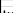nmissingdistinct
4083004
``` Value          I    II   III    IV
Frequency  34825  5141   551   313
Proportion 0.853 0.126 0.013 0.008
```

age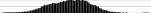nmissingdistinctInfoMeanGmd.05.10.25.50.75.90.95
4083005575160.913.6240.8944.6952.0961.5269.8676.2579.45
lowest : 19.027 19.031 20.289 20.781 20.969 , highest: 92.953 95.000 96.547 108.000 110.000
pulse: Heart Rate beats/min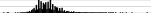nmissingdistinctInfoMeanGmd.05.10.25.50.75.90.95
4083001660.99975.4119.55 50 55 62 73 86 98107
lowest : 0 1 6 8 9 , highest: 200 205 210 220 246
sysbp: Systolic Blood Pressure mmHg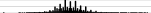nmissingdistinctInfoMeanGmd.05.10.25.50.75.90.95
4083002090.99912926.56 92100112130144160170
lowest : 0 30 32 36 40 , highest: 270 274 275 276 280
miloc: MI Location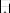nmissingdistinct
4083003
``` Value      Inferior    Other Anterior
Frequency     23495     1435    15900
Proportion    0.575    0.035    0.389
```

pmi: Previous MI
nmissingdistinct
4083002
``` Value         no   yes
Frequency  34104  6726
Proportion 0.835 0.165
```

tx: Tx in 3 groups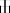nmissingdistinct
4083003
``` Value      SK+tPA     SK    tPA
Frequency   10320  20162  10348
Proportion  0.253  0.494  0.253
```

## Base Risk Model

A simplified covariate-adjusted risk model as developed by Steyerberg is fitted below. For simplicity when later interacting baseline variables with treatment, the important age by Killip class interaction is omitted. Pulse rate is modeled using a linear spline with a knot at 50 beats/minute.

Code
``````require(rms)
options(prType='html')
f <- lrm(day30 ~ tx + age + Killip + pmin(sysbp, 120) +
lsp(pulse, 50) + pmi + miloc, data=gusto,
eps=0.005, maxit=30)
f``````

Logistic Regression Model

``` lrm(formula = day30 ~ tx + age + Killip + pmin(sysbp, 120) +
lsp(pulse, 50) + pmi + miloc, data = gusto, eps = 0.005,
maxit = 30)
```
Model Likelihood
Ratio Test
Discrimination
Indexes
Rank Discrim.
Indexes
Obs 40830 LR χ2 4143.09 R2 0.243 C 0.820
0 37979 d.f. 12 R212,40830 0.096 Dxy 0.641
1 2851 Pr(>χ2) <0.0001 R212,7955.8 0.405 γ 0.641
max |∂log L/∂β| 3×10-9 Brier 0.055 τa 0.083
β S.E. Wald Z Pr(>|Z|)
Intercept  -3.4692  0.7095 -4.89 <0.0001
tx=SK   0.0742  0.0513 1.45 0.1483
tx=tPA  -0.1358  0.0609 -2.23 0.0258
age   0.0796  0.0021 37.12 <0.0001
Killip=II   0.6011  0.0511 11.76 <0.0001
Killip=III   1.2092  0.1053 11.49 <0.0001
Killip=IV   1.9292  0.1391 13.87 <0.0001
sysbp  -0.0391  0.0017 -23.40 <0.0001
pulse  -0.0210  0.0141 -1.48 0.1376
pulse’   0.0406  0.0144 2.82 0.0048
pmi=yes   0.4714  0.0486 9.70 <0.0001
miloc=Other   0.3144  0.1162 2.70 0.0068
miloc=Anterior   0.5441  0.0444 12.27 <0.0001

## Semi-Saturated Model

The only way that ORs for treatment can vary in a logistic model is for there to be one or more interactions between baseline covariates and treatment. Here we allow for all interactions with treatment, which will allow ORs, RRs, and ARRs to vary as the data dictate, assuming that three-way interactions are not needed, e.g., the infarct location-specific treatment effect does not depend on age.

Code
``````g <- lrm(day30 ~ tx * (age + Killip + pmin(sysbp, 120) +
lsp(pulse, 50) + pmi + miloc), data=gusto,
eps=0.005, maxit=60)
print(g, coefs=FALSE)``````
Logistic Regression Model
``` lrm(formula = day30 ~ tx * (age + Killip + pmin(sysbp, 120) +
lsp(pulse, 50) + pmi + miloc), data = gusto, eps = 0.005,
maxit = 60)
```
Model Likelihood
Ratio Test
Discrimination
Indexes
Rank Discrim.
Indexes
Obs 40830 LR χ2 4159.64 R2 0.244 C 0.821
0 37979 d.f. 32 R232,40830 0.096 Dxy 0.641
1 2851 Pr(>χ2) <0.0001 R232,7955.8 0.405 γ 0.642
max |∂log L/∂β| 4×10-11 Brier 0.055 τa 0.083

The likelihood ratio test is the gold-standard frequentist method for testing for added value of the treatment interactions, i.e., whether the treatment ORs are constant over covariate settings. By using a chunk test to test whether any of the baseline variables interact with treatment, this method has a perfect multiplicity adjustment in the frequentist sense.

Code
``lrtest(f, g)``
``````
Model 1: day30 ~ tx + age + Killip + pmin(sysbp, 120) + lsp(pulse, 50) +
pmi + miloc
Model 2: day30 ~ tx * (age + Killip + pmin(sysbp, 120) + lsp(pulse, 50) +
pmi + miloc)

L.R. Chisq       d.f.          P
16.552157  20.000000   0.681833 ``````

We see no evidence to suggest HTE for t-PA or SK effects. We can also use AIC to assess whether allowing for interactions will likely result in better patient-specific outcome predictions.

Code
``c(AIC(f), AIC(g))``
`` 16558.31 16581.76``

The simpler model has a lower (better) AIC, indicating that treatment interactions are not strong enough to overcome the overfitting that allowing for them would entail. In other words, the interactions are likely more noise than signal.

We can also compare the two models by computing the relative explained variation on the risk scale as detailed here.

Code
``````rexv <- var(predict(f, type='fitted')) /
var(predict(g, type='fitted'))
rexv``````
`` 0.9959584``

From this we see that even without penalizing for overfitting, all the treatment interactions account for only 0.004 of the predictive information. The no-interaction logit-additive model that assumes constancy of treatment ORs is at least 0.996 adequate on a scale from 0 to 1.

## Back to the Difficulty of the Task

Covariate-specific treatment effects are combinations of main effects and interaction effects, and one needs evidence that the interaction effect is non-zero before covariate-specific relative efficacy is interesting. As an example of how difficult it is to estimate differential treatment effects (here, double differences on the logit scale, or ratios of odds ratios), let’s temporarily re-fit the logistic model including only a single treatment interaction—with location of infarct. Consider just inferior vs. anterior infarcts, which are the dominant categories and are not too imbalanced.

We estimate the double difference in log odds for t-PA vs. SK in anterior infarcts minus t-PA vs. SK in inferior infarcts. Then we get the frequency-weighted overall treatment effect in these two groups, which is similar to dropping the interaction from the model.

Code
``````i <- lrm(day30 ~ tx * miloc + age + Killip + pmin(sysbp, 120) +
lsp(pulse, 50) + pmi, data=gusto,
eps=0.005, maxit=30)
contrast(i, list(tx='tPA', miloc='Inferior'),
list(tx='SK',  miloc='Inferior'),
list(tx='tPA', miloc='Anterior'),
list(tx='SK',  miloc='Anterior'))``````
``````    Contrast      S.E.       Lower     Upper    Z Pr(>|z|)
11 0.1546134 0.1081337 -0.05732489 0.3665516 1.43   0.1528

Confidence intervals are 0.95 individual intervals``````
Code
``````w <- with(gusto, c(sum(miloc=='Inferior'), sum(miloc=='Anterior')))
contrast(i, list(tx='tPA', miloc=c('Inferior', 'Anterior')),
list(tx='SK',  miloc=c('Inferior', 'Anterior')),
type='average', weights=w)``````
``````    Contrast       S.E.     Lower       Upper     Z Pr(>|z|)         var
1 -0.1838079 0.05588118 -0.293333 -0.07428276 -3.29    0.001 0.003122706

Confidence intervals are 0.95 individual intervals``````

The first contrast (double difference; differential treatment effect) has a standard error that is twice that of the second contrast (treatment effect averaged over the two infarct locations), and only the latter overall treatment effect has evidence for being non-zero (i.e., a benefit of t-PA).

## Penalization

Bayesian modeling of HTE uses hierarchical random effects with shrinkage of interaction effects towards zero, e.g., shrinkage of “subgroup” effects towards the grand mean treatment effect. Borrowing information in this fashion is an optimal way to get covariate-specific treatment effect estimates. The frequentist counterpart that handles mixtures of continuous and categorical baseline variables is penalized maximum likelihood estimation. We will leave the main effects unpenalized, favoring an additive (in the log odds) model with many fewer parameters but allowing the completely unpenalized model to also have a chance at winning, and find the penalty for interaction terms that optimizes the effective AIC, i.e., the AIC computed using the effective degrees of freedom. Note that the `pentrace` function uses the likelihood ratio χ2 scale for AIC unlike the traditional AIC formula, i.e., here bigger AIC is better.

Code
``````h <- update(g, x=TRUE, y=TRUE)
p <- pentrace(h,
list(simple=0,
interaction=1000*
c(1, 5, 10, 15, 20, 30, 40, 100, 200, 500, 1000)),
maxit=30)
p``````
``````
Best penalty:

simple interaction       df
0       1e+06 12.03211

simple interaction       df      aic      bic    aic.c
0        1000 20.33720 4111.002 3935.753 4110.981
0        5000 15.75213 4115.700 3979.961 4115.687
0       10000 14.32236 4117.036 3993.618 4117.025
0       15000 13.69510 4117.613 3999.600 4117.603
0       20000 13.33778 4117.937 4003.003 4117.928
0       30000 12.94300 4118.289 4006.757 4118.280
0       40000 12.72881 4118.477 4008.791 4118.469
0      100000 12.30905 4118.837 4012.768 4118.829
0      200000 12.15779 4118.963 4014.197 4118.955
0      500000 12.06394 4119.040 4015.083 4119.032
0     1000000 12.03211 4119.066 4015.383 4119.058``````
Code
``````h <- update(g, penalty=list(simple=0, interaction=30000),
maxit=30, eps=0.005)
effective.df(h)``````
``````
Original and Effective Degrees of Freedom

Original Penalized
All                            32     12.94
Simple Terms                   11     11.00
Interaction or Nonlinear       21      1.94
Nonlinear                       3      1.05
Interaction                    20      0.94
Nonlinear Interaction           2      0.05``````

The following plots show first the main effect coefficients (with interaction effects penalized vs. not penalized), and second the interaction effect coefficients. Note that main effect coefficients can change just because of penalizing interactions, just as removing interactions from models can greatly change main effects.

Code
``````ia <- grep('\\*', names(coef(g)))
x <- coef(g)[-c(1,ia)]; y <- coef(h)[-c(1,ia)]
r <- range(c(x, y))
plot(x, y,
xlab='Unpenalized Coefficients',
ylab='Penalized Coefficients',
main='Main Effects', xlim=r, ylim=r)
abline(a=0, b=1, col=gray(.85))``````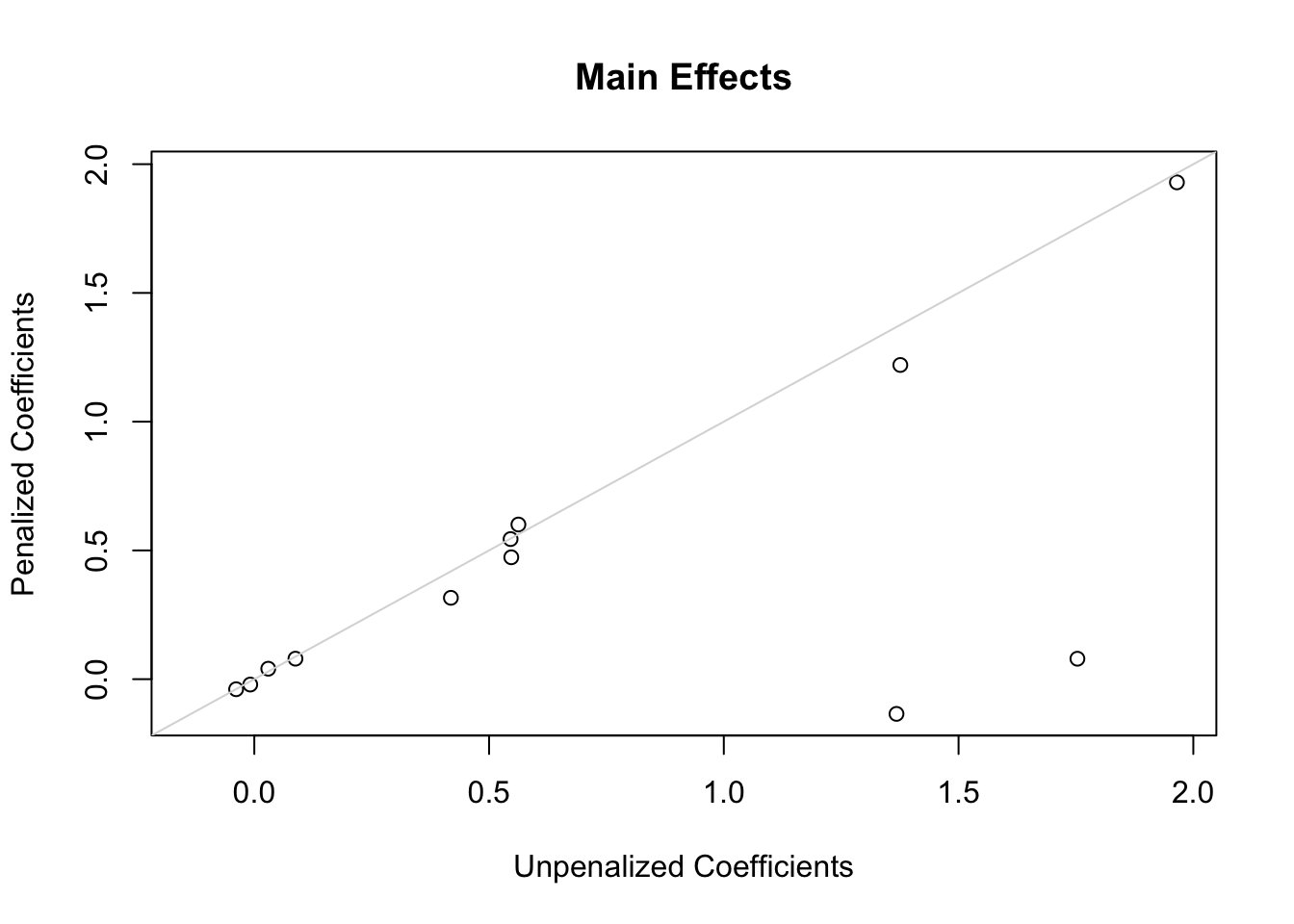Code
``````x <- coef(g)[ia]; y <- coef(h)[ia]
r <- range(c(x, y))
plot(x, y,
xlab='Unpenalized Coefficients',
ylab='Penalized Coefficients',
main='Interaction Parameters', xlim=r, ylim=r)
abline(a=0, b=1, col=gray(.85))``````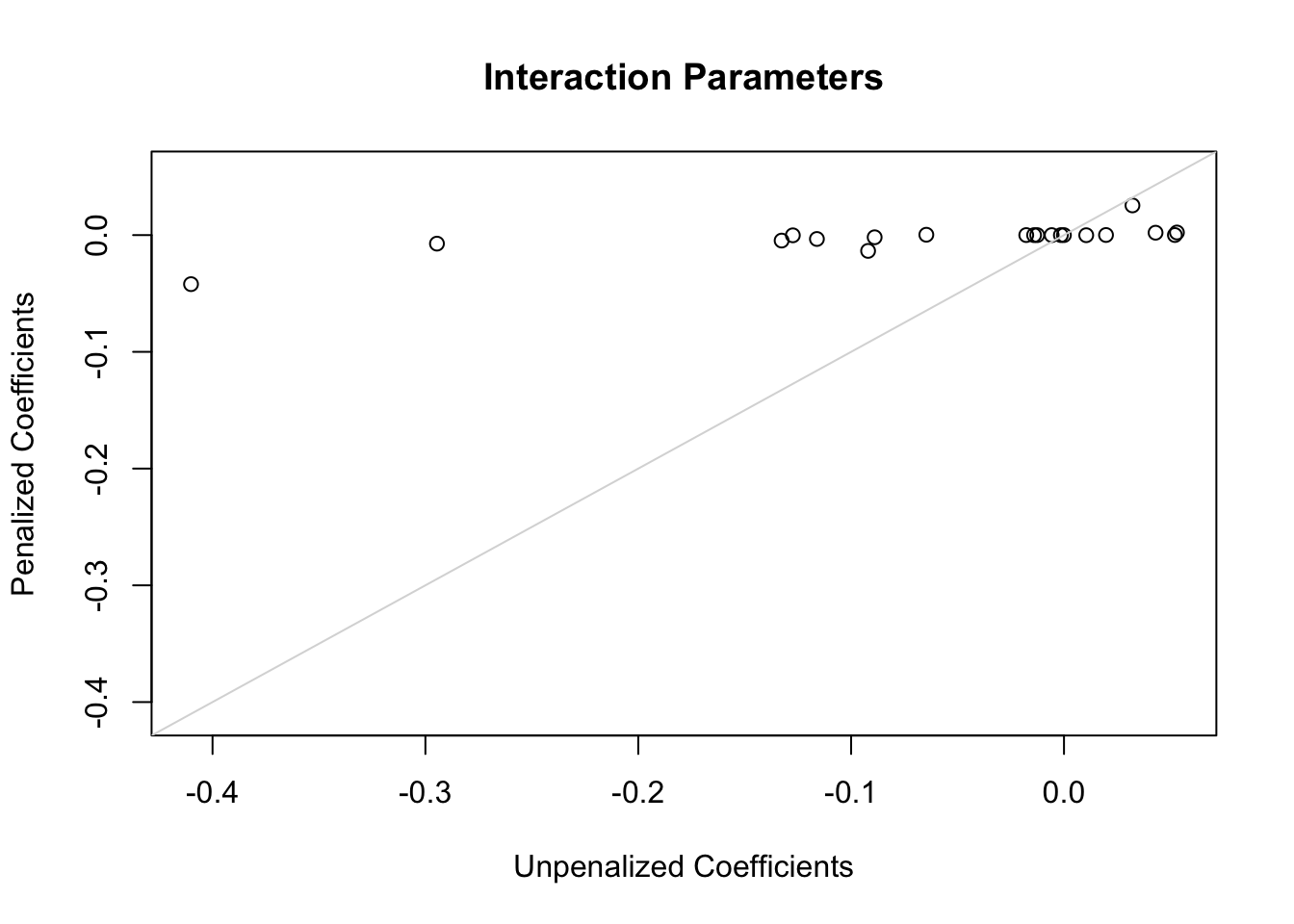Of the penalties studied, the best penalty by both AIC and corrected AIC is 10^{6} which effectively makes the model estimate 12.03 parameters instead of the original 12 parameters. The optimum penalty is really infinity, consistent the the likelihood ratio test for presence of any interactions with treatment. To give the benefit of the doubt we will use a penalty of 30000 to achieve an effective d.f. for interactions of 0.94.

### Using the Penalized Semi-Saturated Model to Estimate Distributions of Various Effects

Even though there is no statistical evidence for HTE (i.e., interactions with treatment on an appropriate scale), we will still allow for all interactions, to give the benefit of the doubt. But we are penalizing the interaction parameters closer to what will cross-validate.

Let’s start with what I believe is the best way to communicate results to an individual patient: estimating the absolute risk of mortality under two treatment options, without hinting how the patient should summarize the two estimates (absolute vs. absolute difference, etc.). The baseline covariate values for one patient are listed below.

Code
``````d <- data.frame(tx=c('SK', 'tPA'), Killip='II', age=55, pulse=40, sysbp=100,
miloc='Inferior', pmi='no')
p <- predict(h, d, type='fitted')
names(p) <- c('SK', 't-PA')
p``````
``````        SK       t-PA
0.04043122 0.03302851 ``````

Now turn to estimating treatment effects for all the patients in the RCT. To estimate patient-specific treatment effects on various scales, we estimate risks of dying within 30 days for each patient with their own covariate settings (baseline covariate values). This is done first with treatment set to SK, no matter which treatment the patient actually received. Then predictions are done setting treatment to t-PA.

Code
``````d <- gusto
d\$tx <- 'SK'
psk  <- predict(h, d, type='fitted')
d\$tx <- 'tPA'
ptpa <- predict(h, d, type='fitted')
k  <- c(5,10,15,20,50,100,200,400,800,1600,3200,20000)
pl <- c(0, quantile(psk, 0.99))
j  <- pmax(psk, ptpa) <= pl
co <- gray.colors(10, 0.75, 0)
ggfreqScatter(psk[j], ptpa[j], cuts=k, fcolors=co,
xlab='P(death|SK)', ylab='P(death|t-PA)')``````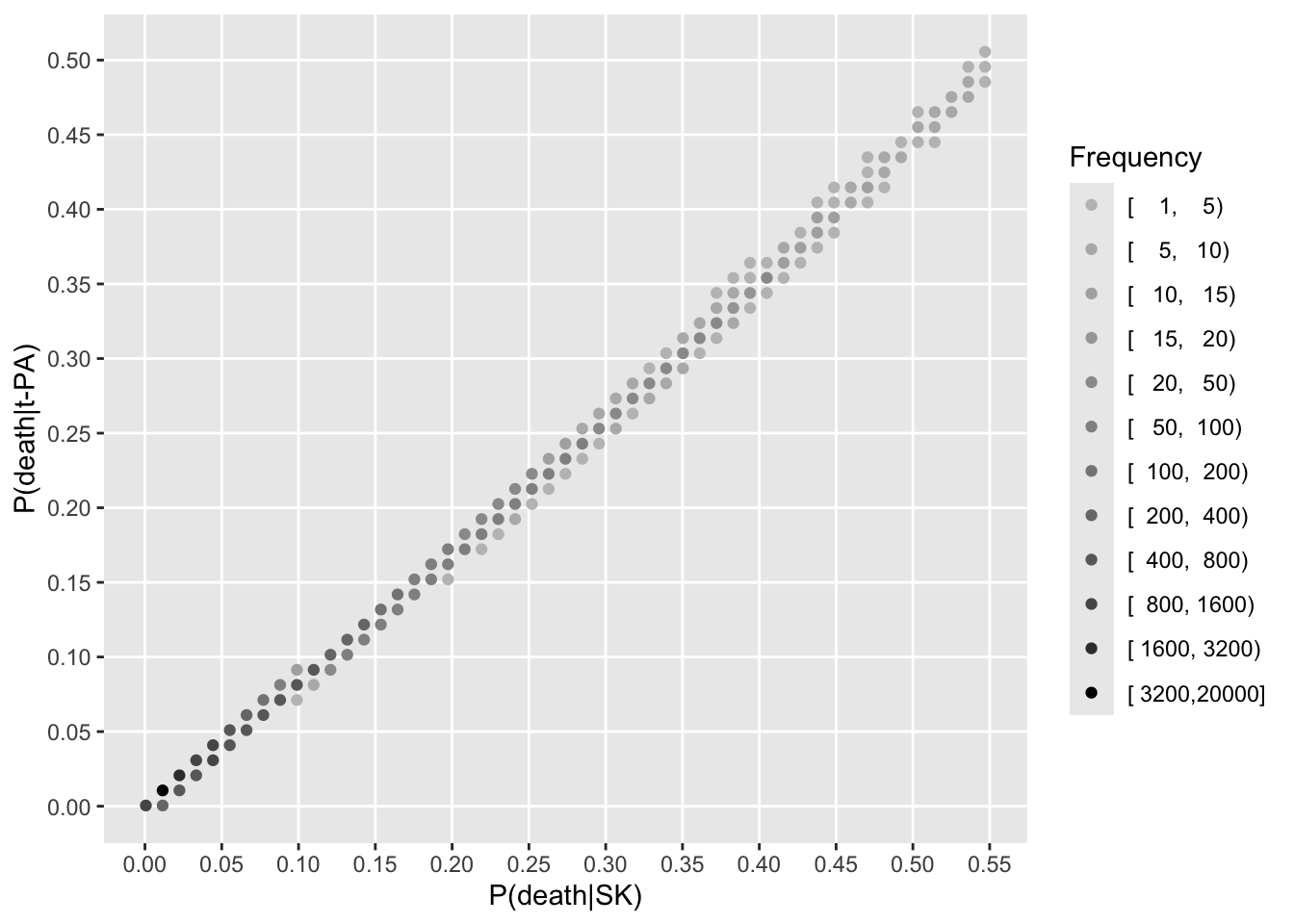Here is the distribution of estimated ARR across all patient types.

Code
``````dif <- psk - ptpa
s   <- c(mean=mean(dif), median=median(dif))
s``````
``````       mean      median
0.011224041 0.006971595 ``````
Code
``````histSpike(dif, bins=seq(-0.02, 0.1, by=0.001), xlim=c(-0.02, 0.1),
xlab='ARR with t-PA', minimal=TRUE)
arrows(s, rep(-0.17, 2), s, rep(-.05, 2), xpd=NA, length=.05) # -0.04 -0.01 for full size``````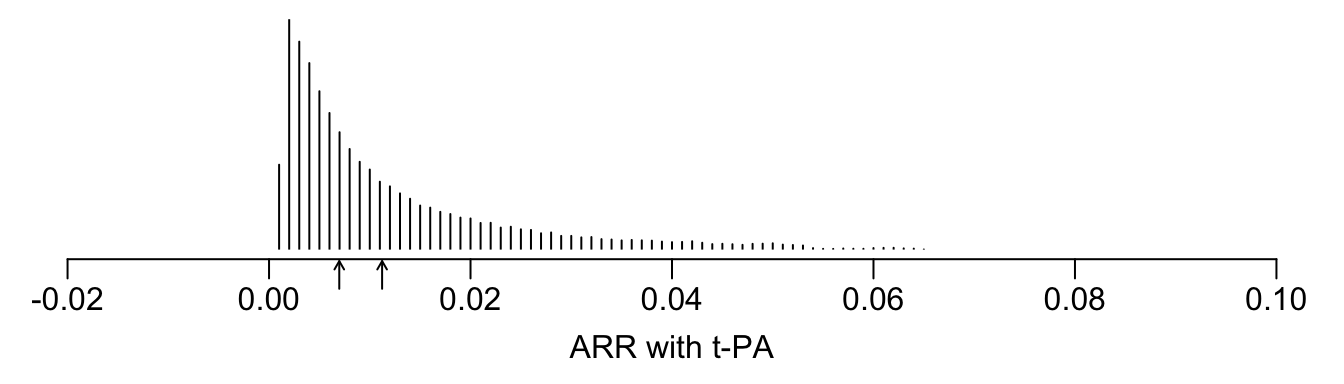And the distribution of estimated RR.

Code
``````histSpike(ptpa / psk, xlim=c(.75, 1), minimal=TRUE,
xlab='RR t-PA/SK')``````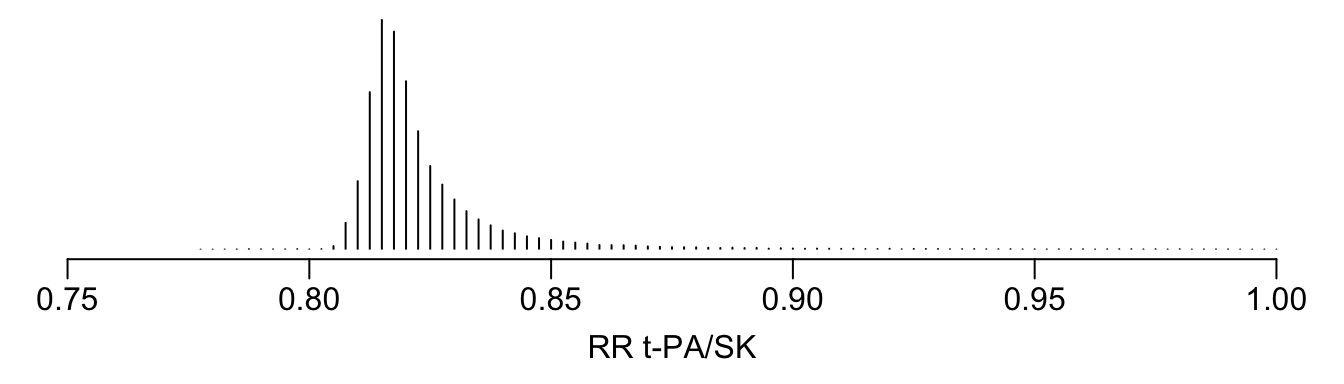And finally the distribution of estimated ORs.

Code
``````or <- exp(qlogis(ptpa) - qlogis(psk))
histSpike(or, xlim=c(.75, 1), minimal=TRUE, xlab='OR')``````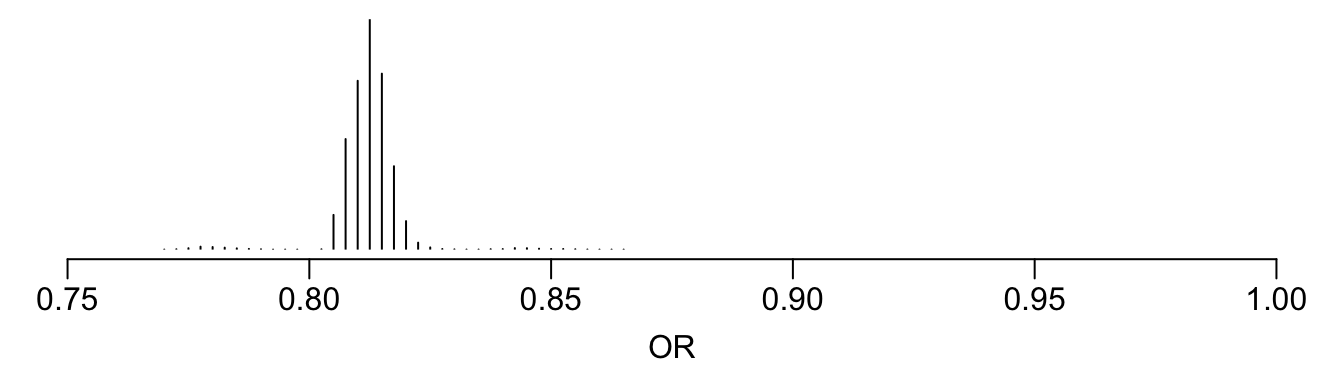The following scatterplot shows the relationship between estimated RRs and estimate ORs.

Code
``ggfreqScatter(or, ptpa / psk, cuts=k, xlab='OR', ylab='RR', fcolors=co)``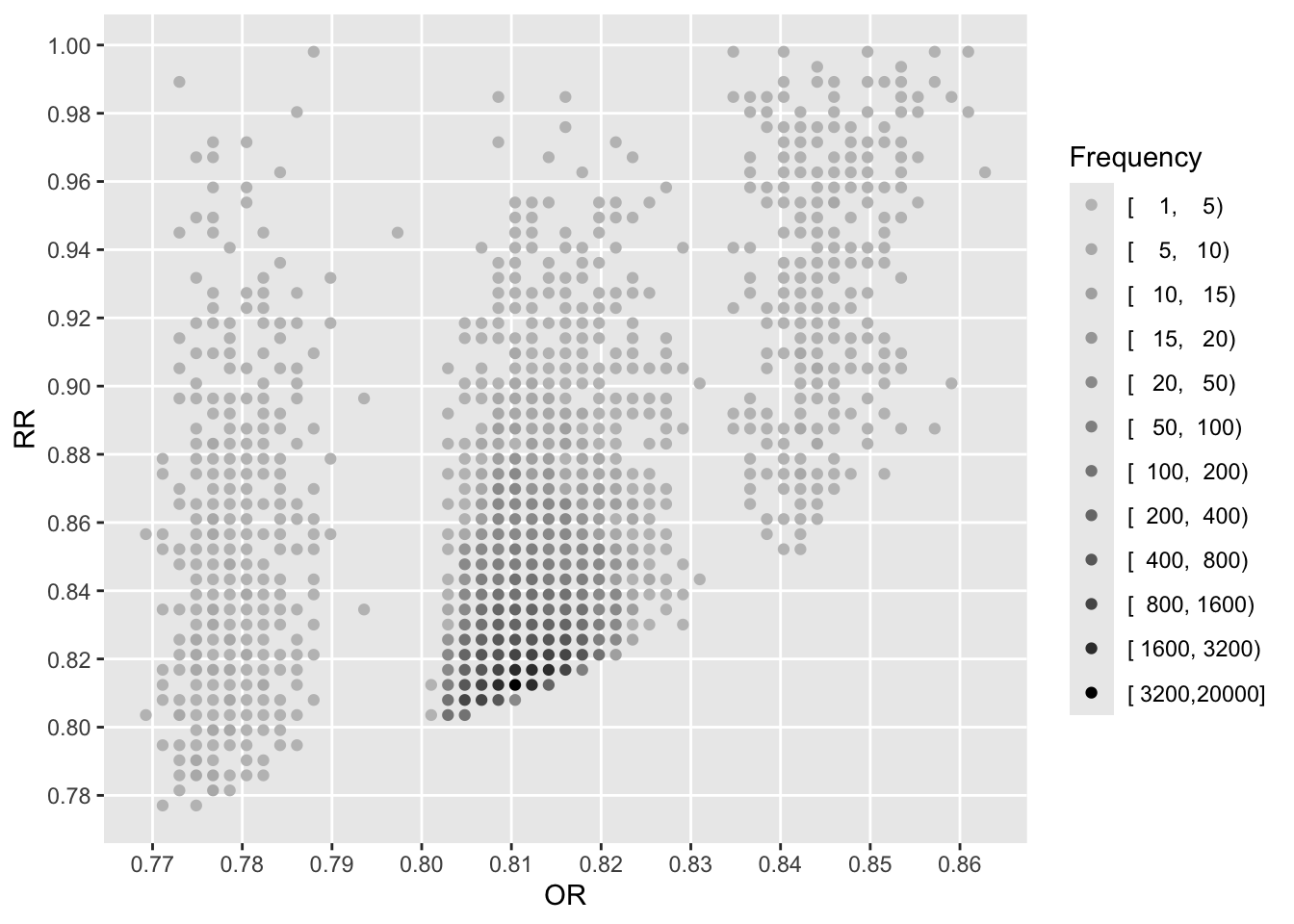See that the variation in absolute or relative risk reduction is large, but adjusted odds ratios for t-PA vs. SK have a very narrow distribution. If we relied on the formal interaction test, this distribution would have a single value.

To see how the various measures depend on baseline risk, see the following graphs.

Code
``````xl <- 'Risk Under Control Treatment (SK)'
j <- psk <= pl
ggfreqScatter(psk[j], psk[j] - ptpa[j], cuts=k, xlab=xl, ylab='ARR', fcolors=co)``````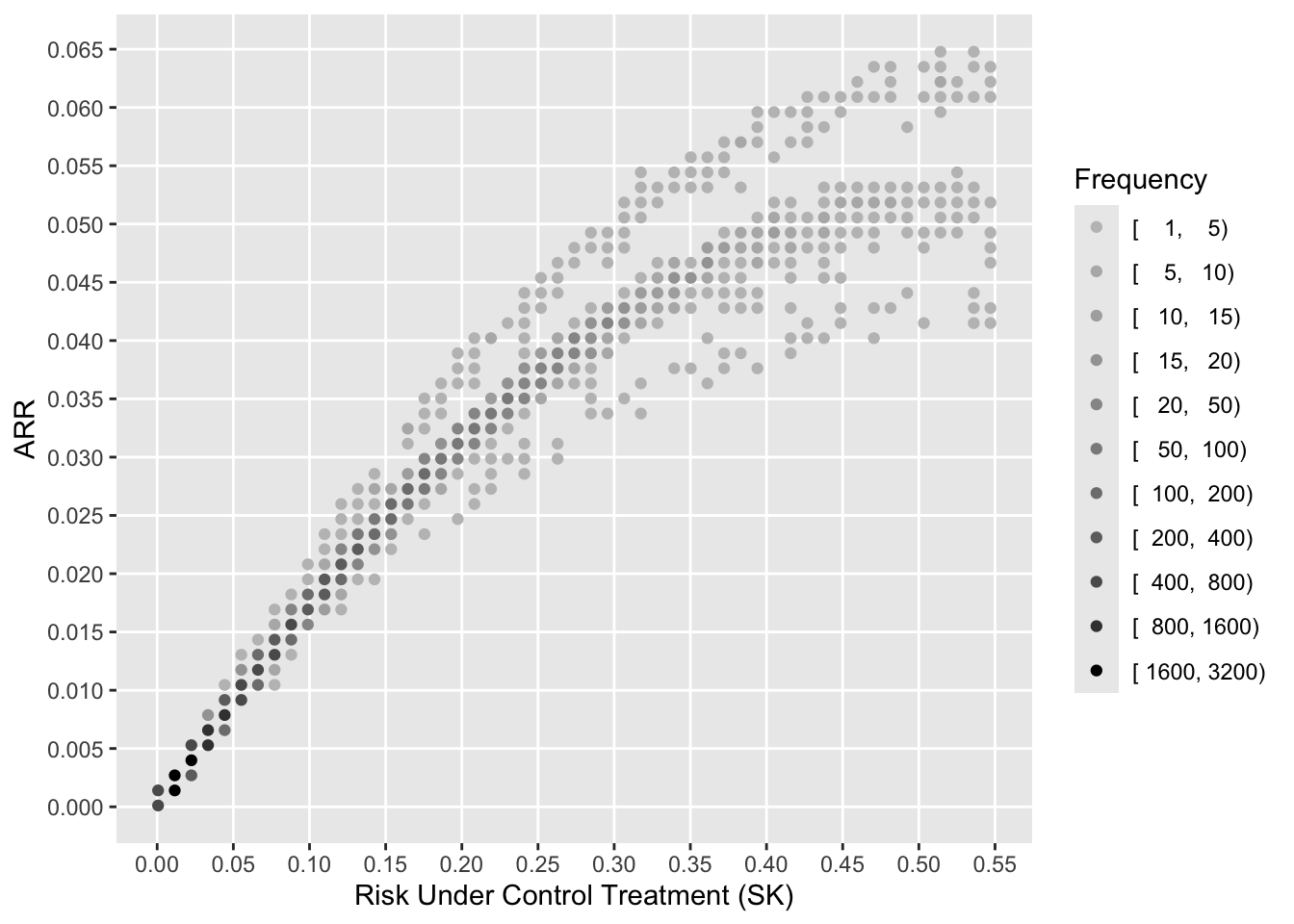Code
``ggfreqScatter(psk[j], ptpa[j] / psk[j], cuts=k, xlab=xl, ylab='RR', fcolors=co)``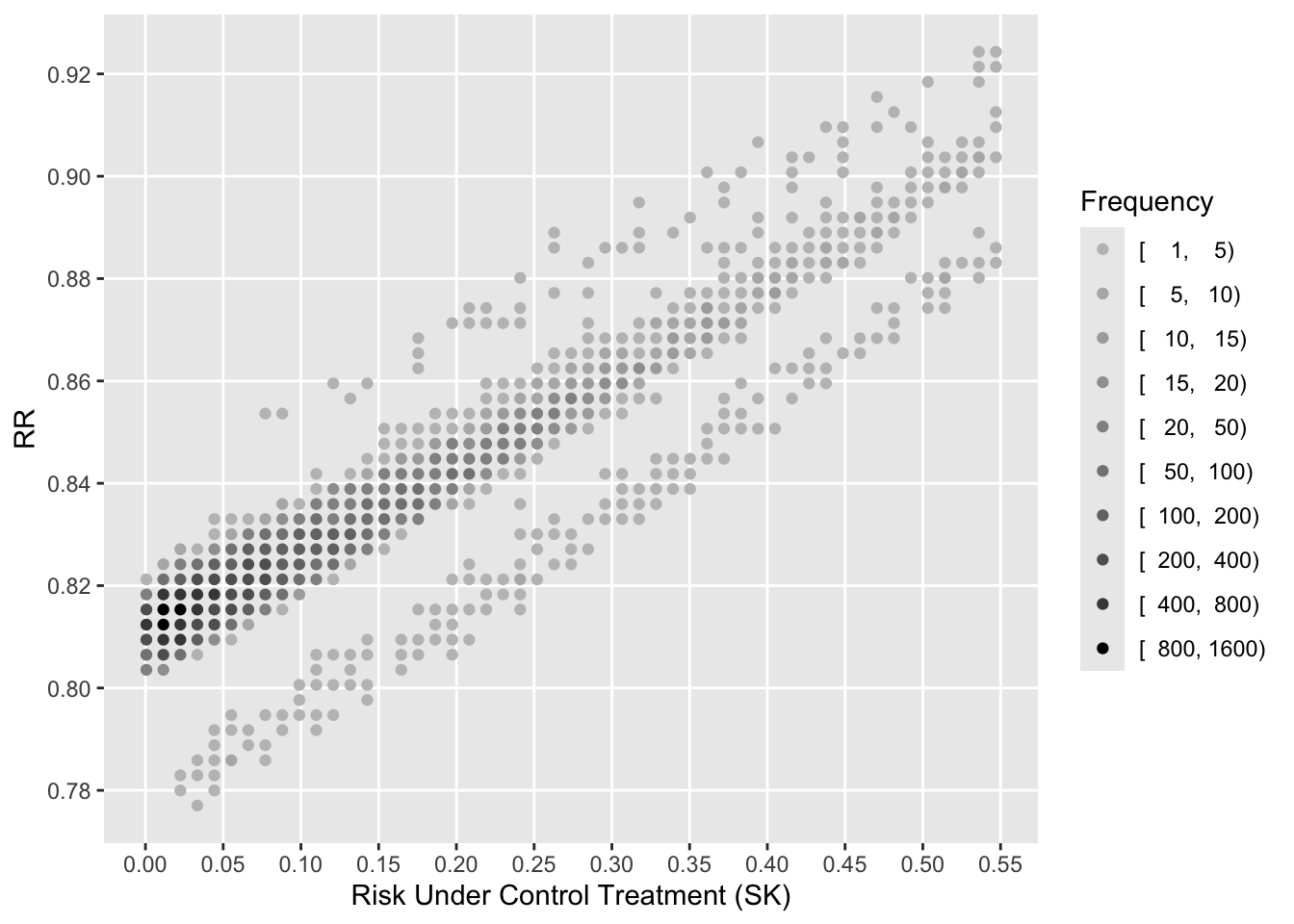Code
``ggfreqScatter(psk[j], or[j], cuts=k, xlab=xl, ylab='OR', fcolors=co)``